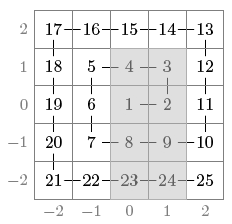Code Submission Evaluation System Login

# BOI 2016, day 1

 Start: 2016-05-12 09:00:00 End: 2016-05-12 14:00:00

CSES - BOI 2016, day 1 - SpiralCSES - Spiral

## Spiral

 Time limit: 1.50 s Memory limit: 256 MB

A grid of size $(2n+1)\times(2n+1)$ has been constructed as follows. Number $1$ has been placed in the center square, number $2$ has been placed to the right of it, and the following numbers have been placed along the spiral counterclockwise.

Your task is to calculate answers for $q$ queries where the sum of numbers in an rectangular region in the grid is requested (modulo $10^9+7$). For example, in the following grid $n=2$ and the sum of numbers in the gray region is $74$:Input

The first input line contains two integers $n$ and $q$: the size of the grid and the number of queries.

After this, there are $q$ lines, each containing four integers $x_1$, $y_1$, $x_2$ and $y_2$ ($-n \le x_1\le x_2 \le n$, $-n \le y_1 \le y_2 \le n$). This means that you should calculate the sum of numbers in a rectangular region with corners $(x_1, y_1)$ and $(x_2, y_2)$.

Output

You should output the answer for each query (modulo $10^9+7$).

Example

Input:
2 3 0 -2 1 1 -1 0 1 0 1 2 1 2

Output:
74 9 14

In all subtasks $1 \le q \le 100$.

• $1 \le n \le 1000$
• $1 \le n \le 10^9$
• $x_1=x_2$ and $y_1=y_2$
• $1 \le n \le 10^5$
• $1 \le n \le 10^9$
• $x_1=y_1=1$
• $1 \le n \le 10^9$